﻿ 长江经济带出口集装箱运输网络优化设计
«上一篇文章快速检索 高级检索

 哈尔滨工程大学学报2018, Vol. 39Issue (6): 990-997  DOI: 10.11990/jheu.2017030630

### 引用本文GONG Zhiguang, WANG Yang, YANG Zhongzhen. Optimization of transport network for export containers in Yangtze River economic belt[J]. Journal of Harbin Engineering University, 2018, 39(6), 990-997. DOI: 10.11990/jheu.201703063.### 文章历史

Optimization of transport network for export containers in Yangtze River economic belt
GONG Zhiguang, WANG Yang, YANG ZhongzhenCollege of transportation Engineering, Dalian Maritime University, Dalian 116026, China
Abstract: To minimize transportation cost, a mathematical expression of a transport network comprising seaways, waterways, land, and transshipment system was given. By considering the navigation ability of different waterways in Yangtze River, the development of the harbors along the river, and the international logistics ability of Yangshan Port, a transport organization optimization model was established on the basis of the path selection behaviors of a cargo owner, to assign the optimum path for the container goods exported from the upstream of Yangtze River. Forty cities in the midstream and downstream regions of Yangtze River were taken as the export start points. Tokyo, Yokohama, Nagoya and Kobeport were taken as the destination ports. By setting two dry ports as the premise, an optimization was carried out for the transport of the exported container for zero to two hub river ports. The results show that the total social cost is lowest for two dry ports and two hub river ports.
Key words: Yangtze River economic belt(YZREB)    export container    multi-mode transport    super network    integrated transport    ship routing    genetic algorithm    user equilibrium

1 长江经济带超级网络构建Download: 图 1 长江经济带出口集装箱运输路径示意图 Fig. 1 Paths of export containers from YZREB

2 两阶段运网优化模型结构 2.1 第一阶段的模型结构

 $\begin{array}{*{20}{c}} {\min {Z_1} = {c^e}\sum\limits_{a \in {A_E}} {{l_a}{q_a}} + {c^s}\sum\limits_{a \in {A_S}} {{q_a}} + }\\ {{c^r}\sum\limits_p {\sum\limits_i {\sum\limits_k {{x_p}{y_{ik}}{l_{pi}}{q_{pi}}} } } }\\ {{c^{wt}}\sum\limits_i {\sum\limits_j {{l_{ij}}{q_{ij}}} } + \sum\limits_k {{z_k}{c^k}} + \mu \sum\limits_{a \in {A_G}} {{t_a}{q_a}} } \end{array}$ (1)

s.t.

 $\sum\limits_k {{z_k}} = 1$ (2)
 $\sum\limits_p {{x_p}} = {n_1}$ (3)
 $\sum\limits_i {{y_{ik}}} = {n_2},i \in {P_1},\forall k \in K$ (4)
 $\begin{array}{*{20}{c}} {{c^k} = 7c_k^{{\rm{fix}}}n_k^{{\rm{ship}}} + {c^{{\rm{bunker1}}}}h_k^{{\rm{bunker1}}}\sum\limits_i {\sum\limits_j {{t_{ij}}} } + }\\ {\left( {\sum\limits_i {\left( {\sum\limits_j {{t_{ij}}} } \right)} + t_i^{{\rm{handle}}} + t_i^{{\rm{wait}}}} \right){c^{{\rm{bunker2}}}}h_k^{{\rm{bunker2}}} + c_k^{{\rm{port}}}} \end{array}$ (5)
 $c_{ij}^k = \left( {{l_{ij}}/\sum\limits_i {\sum\limits_j {{l_{ij}}} } } \right)/\left( {{c^k}/{\rm{Ca}}{{\rm{p}}_k}} \right)$ (6)
 $n_k^{{\rm{ship}}} = {f_k}\left( {\sum\limits_i {\left( {\sum\limits_j {{t_{ij}}} } \right)} + t_i^{{\rm{handle}}} + t_i^{{\rm{wait}}}} \right)/7$ (7)
 ${y_p} \in \left\{ {0,1} \right\},\forall p \in P$ (8)
 ${y_{ik}} \in \left\{ {0,1} \right\},\forall i \in {A_{Wk}},\forall k \in K$ (9)
 ${z_k} \in \left\{ {0,1} \right\},\forall k \in K$ (10)

2.2 第二阶段的模型结构
 $\min {Z_2} = \sum\limits_{a \in {A_G}} {\int_0^{{q_a}} {{c_a}\left( w \right){\rm{d}}w} }$ (11)
 ${\rm{s}}.\;{\rm{t}}.\sum\limits_{r \in R} {f_r^{{\rm{od}}}} = {Q_{{\rm{od}}}},\forall o \in O,\forall d \in D$ (12)
 $\begin{array}{*{20}{c}} {\sum\limits_o {\sum\limits_d {\sum\limits_r {\delta _{a,r}^{{\rm{od}}}f_r^{{\rm{od}}}} } } = {q_a},\forall o \in O,\forall d \in D,}\\ {\forall r \in R} \end{array}$ (13)
 $f_r^{{\rm{od}}} \ge 0,\forall o \in O,\forall d \in D$ (14)

3 实例分析 3.1 数据收集

 $\mu = {V_g} \times i/365$ (23)表 1 江海直达船舶数据 Tab.1 Specification of the river-sea ships

3.2 求解及结果分析

1) 第一阶段模型的求解算法。

① 编码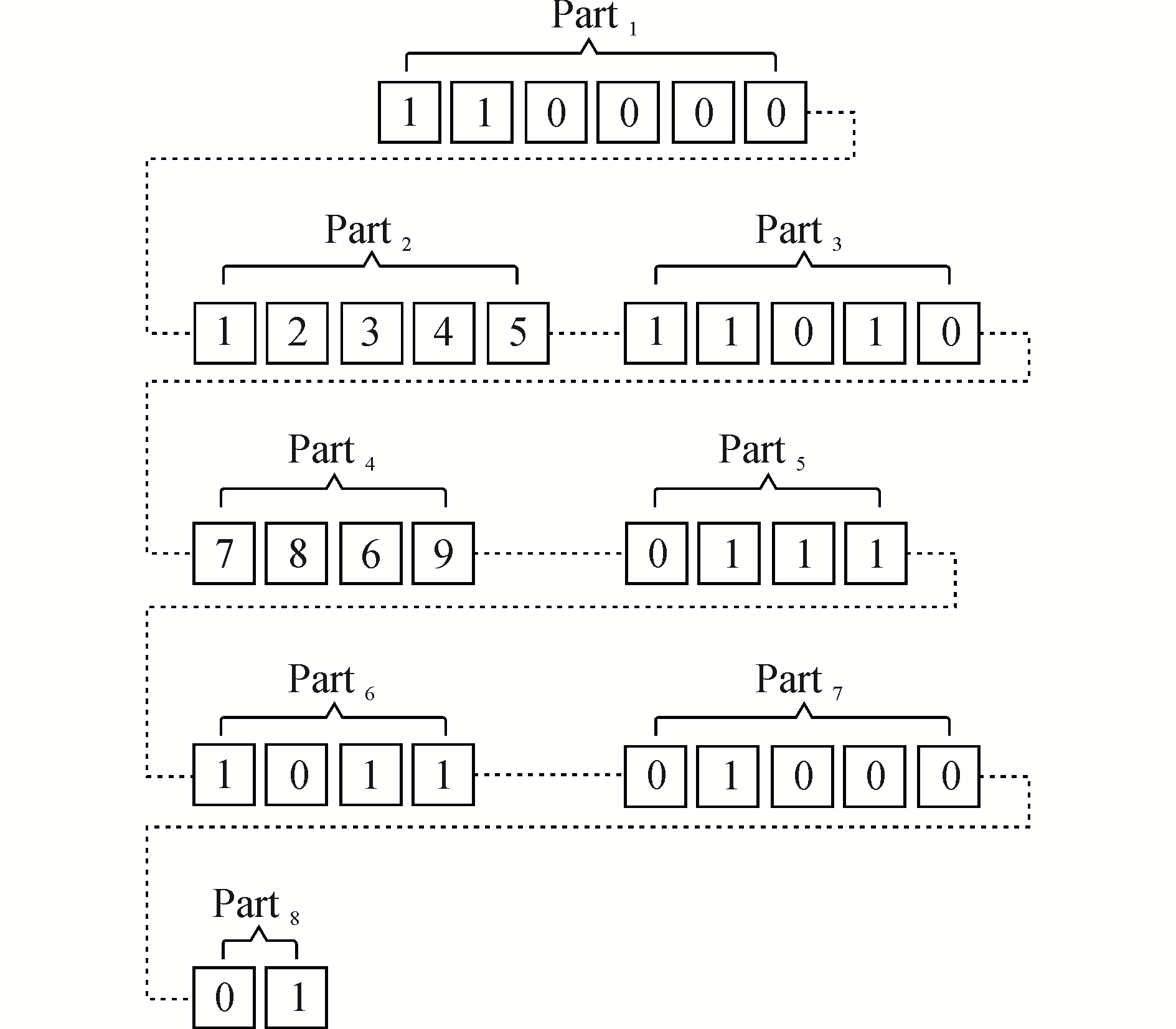Download: 图 2 编码方法 Fig. 2 Coding method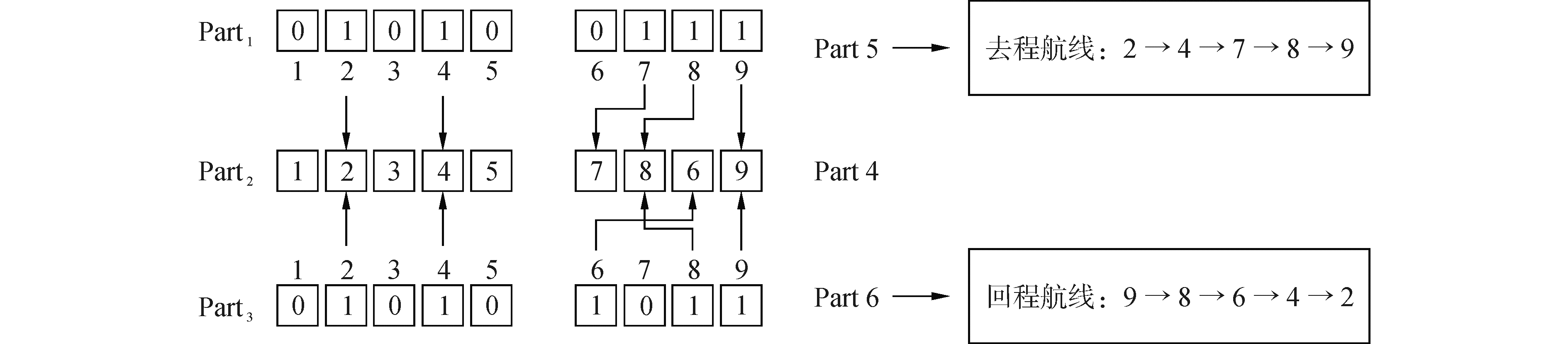Download: 图 3 解码方法 Fig. 3 Decoding method

② 交叉操作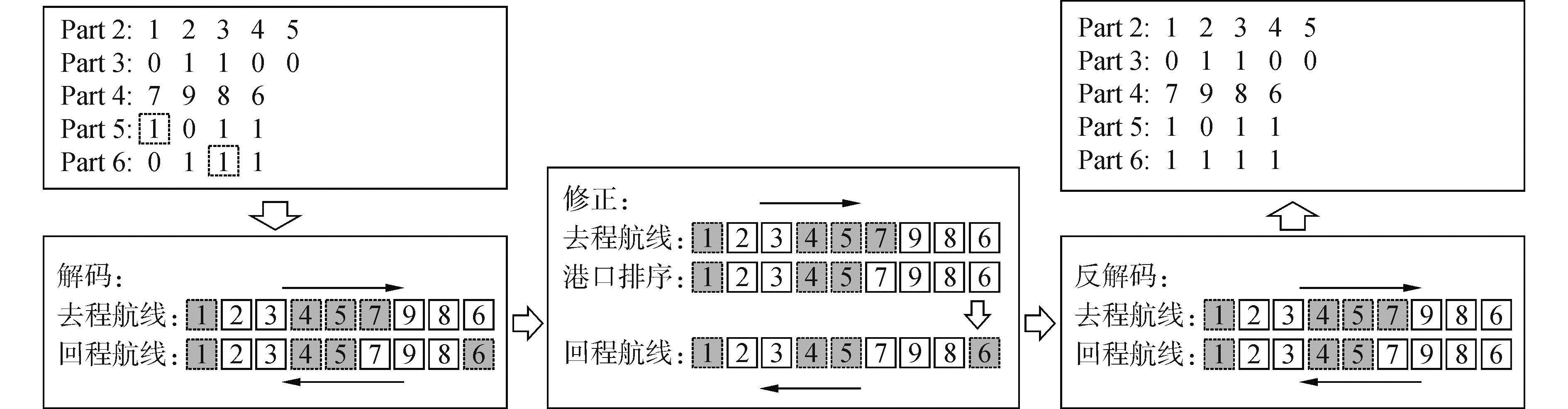Download: 图 4 编码修正方法 Fig. 4 Correct method of genetic code

③ 变异操作

2) 第二阶段模型的求解算法。

3) 第一阶段的结果及分析。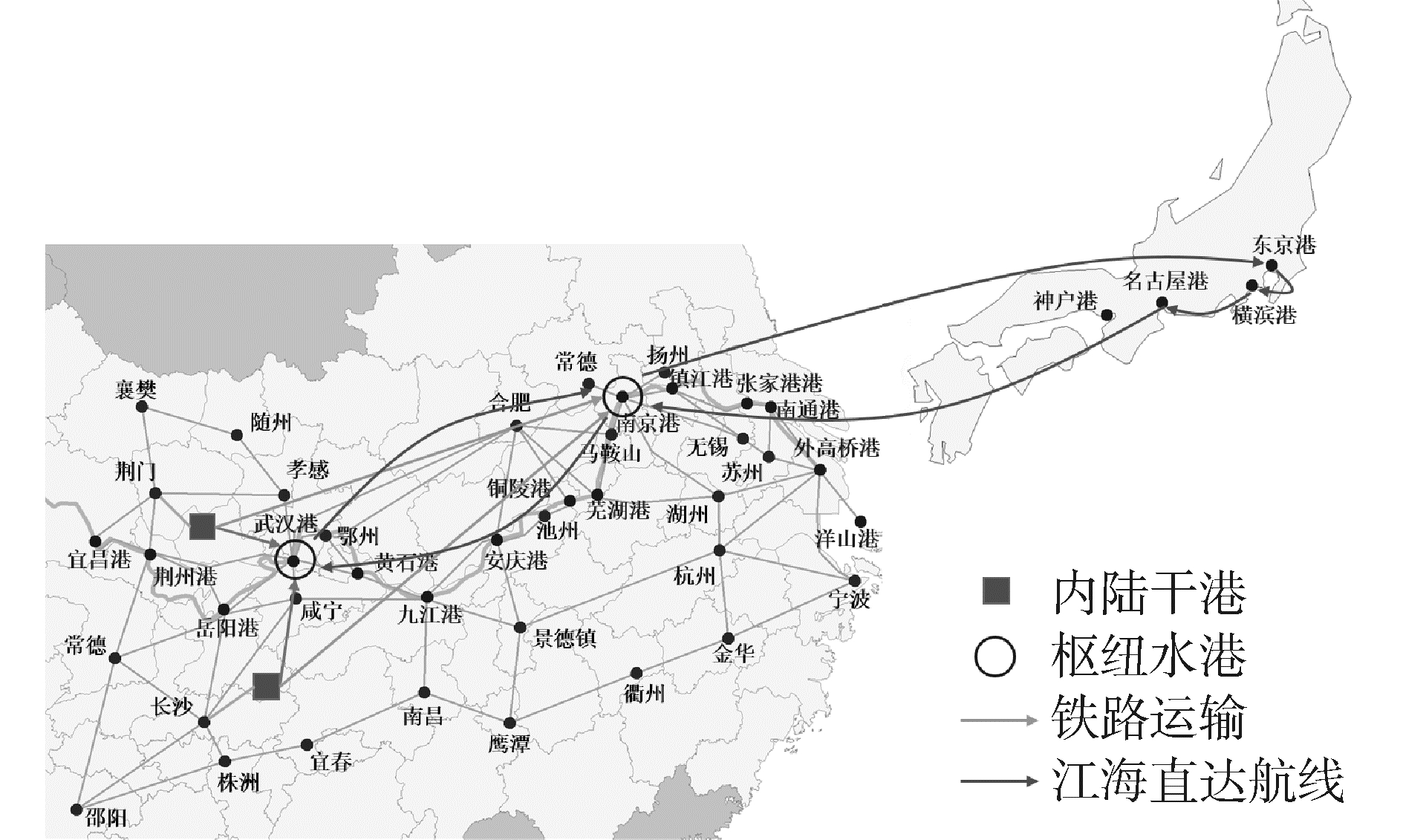Download: 图 5 设置2个干港和2个枢纽河港时的选址与航线情况 Fig. 5 Cases of setting 2 dry ports and 2 hub river ports

4) 第二阶段的结果及分析。

① 干港及其换装量表 2 设置2个枢纽水港时干港的运输情况 Tab.2 Cases of dry ports when setting 2 river hub ports

② 枢纽河港及其江海直达量表 3 两个枢纽河港的运输量 Tab.3 Container volumes in the two hub river portsTEU

③ 河港运输至洋山港的中转量Download: 图 6 设置2个枢纽河港时内河港口的运量 Fig. 6 Volume in inland ports when setting 2 hub river ports
3.3 针对枢纽河港数量的敏感性分析

1) 无枢纽河港(n2=0，干港数量不变，即n1=2)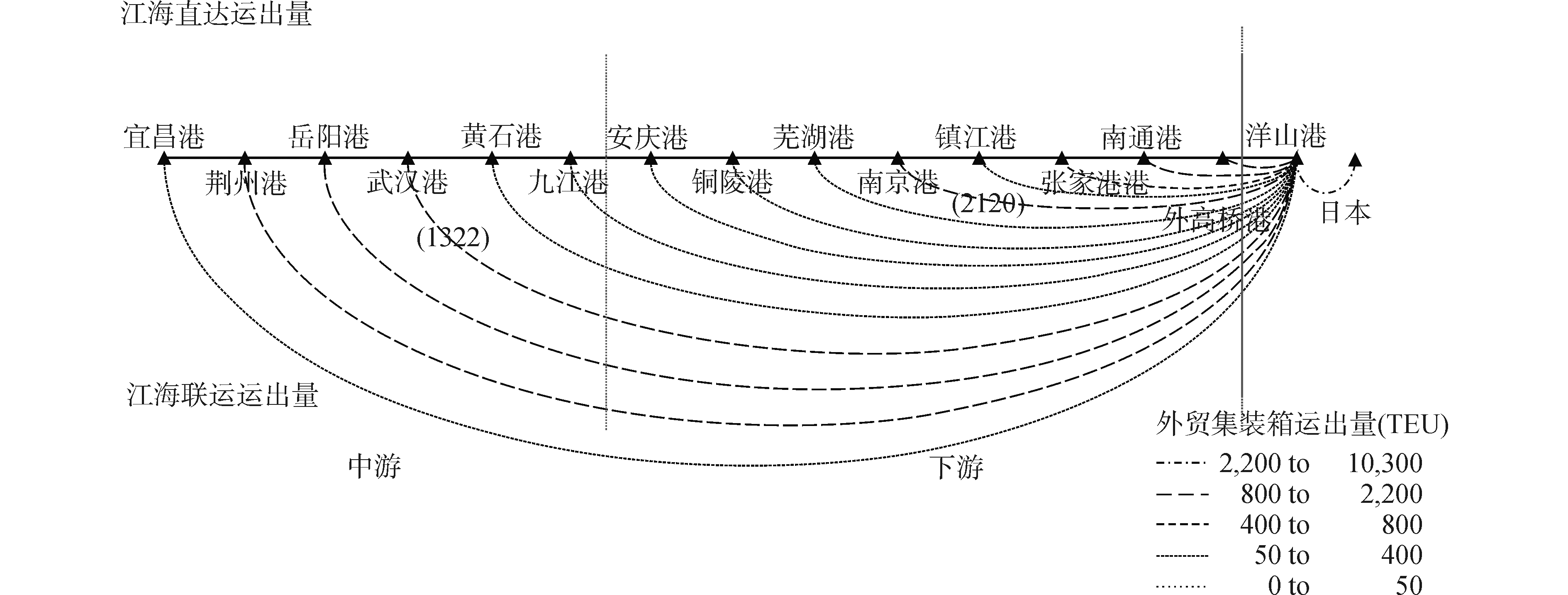Download: 图 7 设置2个干港、0个枢纽河港时内河港口的运量 Fig. 7 Volumes in inland ports when setting 2 dry ports and 0 hub river port

2) 1枢纽河港(n2=1，干港数量不变，即n1=2)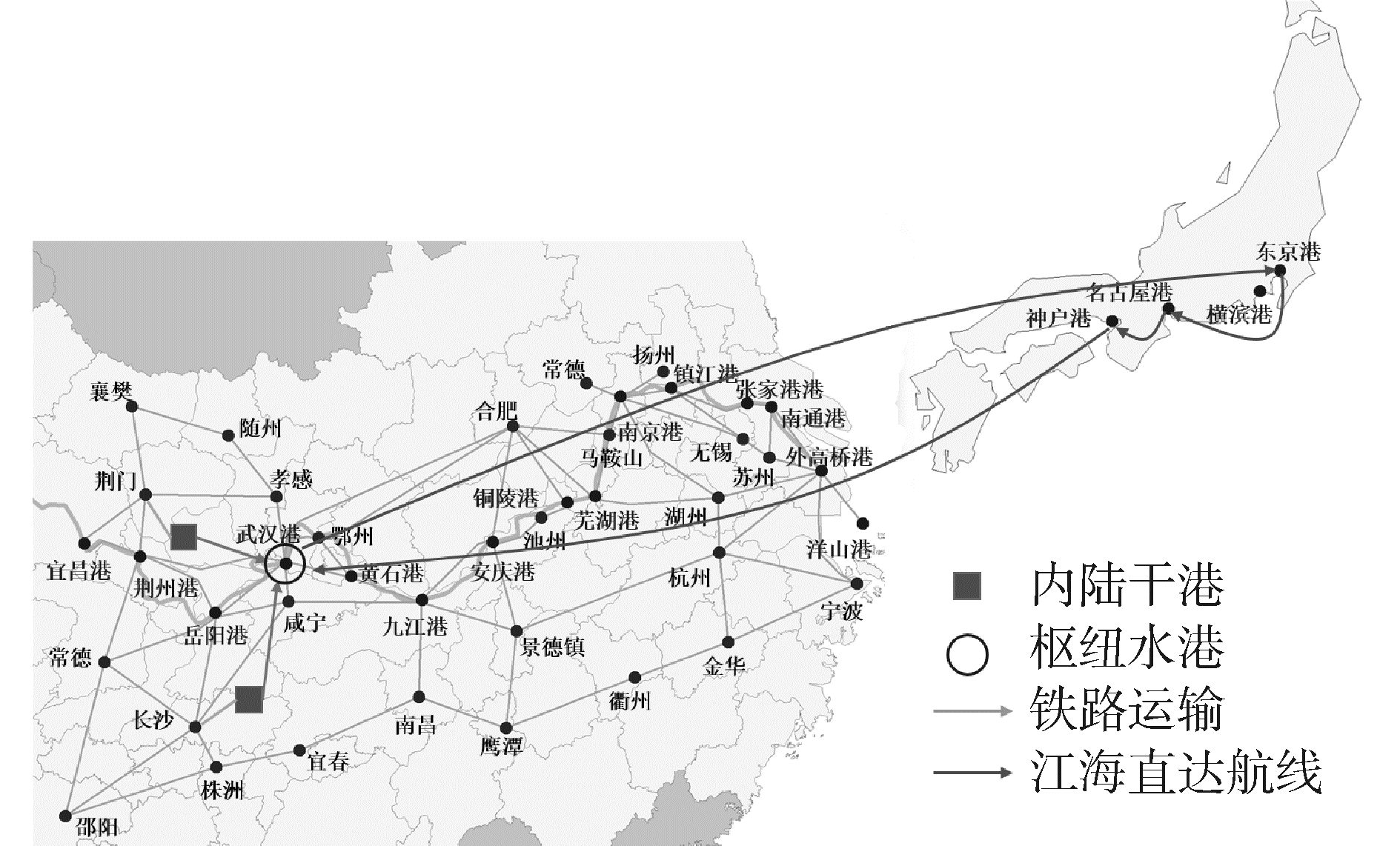Download: 图 8 设置2个干港和1个枢纽河港时的选址与航线情况 Fig. 8 Cases of setting 2 dry ports and 1 hub river port表 4 设置1个枢纽水港时干港的运输情况 Tab.4 Cases of dry ports when setting 1 hub river port TEU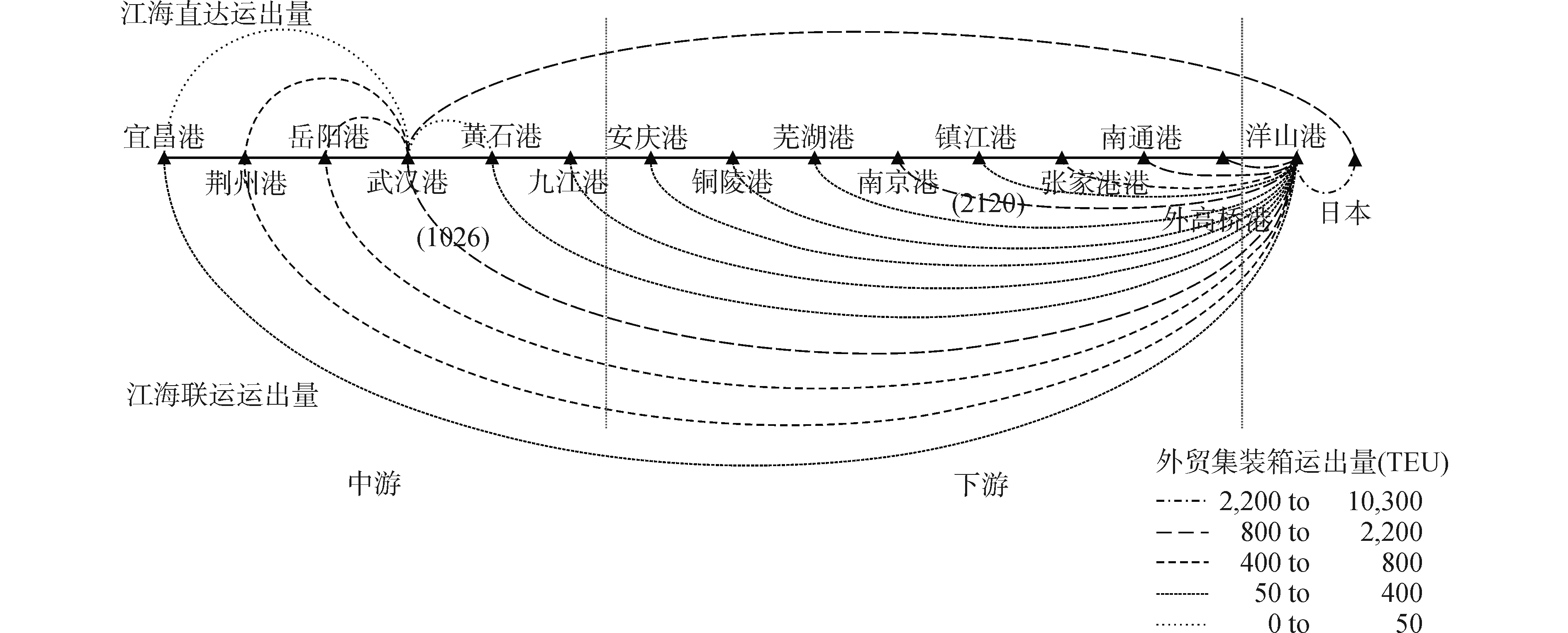Download: 图 9 设置2个干港和1个枢纽水港时内河港口的运量 Fig. 9 Volumes in inland ports when setting 2 dry ports and 1 hub river port
4 结论

1) 干港适合选址在公路枢纽处且距离河港300 km左右的城市；

2) 武汉港和南京港拥有大量出口运输需求，适合作为长江中游和下游的枢纽河港，其中武汉港适合发展江海直达运输，南京港在发展江海直达运输的同时，还应重点发展江海联运模式；

3) 本文提出的模型、理论以及方法对于构建长江经济带综合交通运输枢纽，实施外贸货物的陆-铁-海联运具有重要的参考价值。

  SHINTANI K, IMAI A, NISHIMURA E, et al. The container shipping network design problem with empty container repositioning[J]. Transportation research part E:logistics and transportation review, 2007, 43(1): 39-59. DOI:10.1016/j.tre.2005.05.003 (0)  陈康, 杨忠振. 基于三方博弈的短途班轮航线优化模型[J]. 交通运输工程学报, 2011, 11(6): 74-81, 88. CHEN Kang, YANG Zhongzhen. Optimization model of short-distance liner ship route based on tripartite game[J]. Journal of traffic and transportation engineering, 2011, 11(6): 74-81, 88. (0)  陈康, 郭利泉, 杨忠振. 基于混合航线结构的集装箱航线与空重箱运输综合优化模型[J]. 系统工程理论与实践, 2014, 34(1): 122-128. CHEN Kang, GUO Liquan, YANG Zhongzhen. Optimization model for container ship routing and empty/full container transport in the context of mixed route structure[J]. Systems engineering-theory & practice, 2014, 34(1): 122-128. DOI:10.12011/1000-6788(2014)1-122 (0)  MARAŠ V, LAZIĆ J, DAVIDOVIĆ T, et al. Routing of barge container ships by mixed-integer programming heuristics[J]. Applied soft computing, 2013, 13(8): 3515-3528. DOI:10.1016/j.asoc.2013.03.003 (0)  ZHENG Jianfeng, YANG Dong. Hub-and-spoke network design for container shipping along the Yangtze River[J]. Journal of transport geography, 2016, 55: 51-57. DOI:10.1016/j.jtrangeo.2016.07.001 (0)  KONINGS R, LUDEMA M. The competitiveness of the river-sea transport system:market perspectives on the United Kingdom-Germany corridor[J]. Journal of transport geography, 2000, 8(3): 221-228. DOI:10.1016/S0966-6923(00)00015-6 (0)  SOUTHWORTH F, PETERSON B E. Intermodal and international freight network modeling[J]. Transportation research part C:emerging technologies, 2000, 8(1/2/3/4/5/6): 147-166. (0)  汪传旭. 基于轴-辐运输系统的区域港口群二级物流运输网络优化[J]. 系统工程理论与实践, 2008, 28(9): 152-158. WANG Chuanxu. Optimization of hub-and-spoke based regional port cluster two stage logistics system network[J]. Systems engineering-theory & practice, 2008, 28(9): 152-158. (0)  魏航, 李军, 蒲云. 时变网络下多式联运的最短路径问题研究[J]. 系统工程学报, 2007, 22(2): 205-209. WEI Hang, LI Jun, PU Yun. Study on the multi-modal shortest path in time-varying network[J]. Journal of systems engineering, 2007, 22(2): 205-209. (0)  ARNOLD P, PEETERS D, THOMAS I. Modelling a rail/road intermodal transportation system[J]. Transportation research part E:logistics and transportation review, 2013, 40(3): 255-270. (0)  HANSSEN T E S, MATHISEN T A, JØRGENSEN F. Generalized transport costs in intermodal freight transport[J]. Procedia-social and behavioral sciences, 2012, 54: 189-200. DOI:10.1016/j.sbspro.2012.09.738 (0)  RESAT H G, TURKAY M. Design and operation of intermodal transportation network in the Marmara region of Turkey[J]. Transportation research part E:logistics and transportation review, 2015, 83: 16-33. DOI:10.1016/j.tre.2015.08.006 (0)  宋炳良. 国际集装箱多式联运与东部港口竞争力分析[J]. 中国航海, 2003(3): 61-64. SONG Bingliang. Analysis of international multi-modal transportation and eastern coastal ports competitiveness[J]. Navigation of China, 2003(3): 61-64. (0)  刘腾远. 内贸集装箱多式联运成本分析[J]. 甘肃科学学报, 2013, 25(2): 142-144. LIU Tengyuan. Analysis of the costs of domestic container intermodal[J]. Journal of Gansu sciences, 2013, 25(2): 142-144. (0)  MENG Qiang, WANG Xinchang. Intermodal hub-and-spoke network design:Incorporating multiple stakeholders and multi-type containers[J]. Transportation research part B:methodological, 2011, 45(4): 724-742. DOI:10.1016/j.trb.2010.11.002 (0)